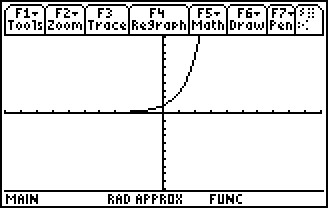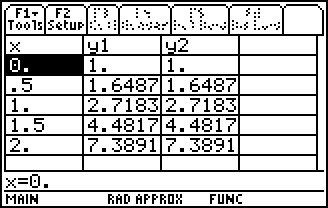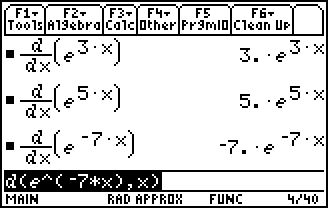# Activities

••• ##### Subject Area

• Math: Calculus: Applications of the Derivative

• ##### Author9-12

45 Minutes

• ##### Device
• TI-89 / TI-89 Titanium

## Exponentially Fast Derivative#### Activity Overview

For ex, students explore the slope of the tangent graphically and use CAS functionality to discover the chain rule for exponential functions.

#### Key Steps

•Part 1 begins with foundational questions that often need reviewed. Graphical reinforcement and self-check multiple-choice questions strength students’ understanding and retention.

•Students will realize that the derivative of ex is unlike the derivative of a polynomial or the power function xn. Students use CAS functionality to discover the rule for the derivative of eu(x).

•Observations of an exponential function and its derivative are made. Inquiry questions are provided for the teacher to draw out deeper understanding. Students will algebraically and numerically confirm observations. Problems are provided to develop proficiency with the Chain Rule as it applies to exponential functions.